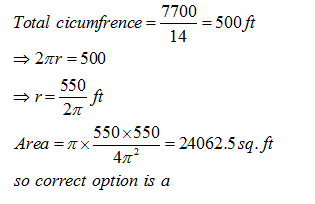• This is an assessment test.
• These tests focus on geometry and mensuration and are meant to indicate your preparation level for the subject.
• Kindly take the tests in this series with a pre-defined schedule.

## Geometry and Mensuration: Test 8

Congratulations - you have completed Geometry and Mensuration: Test 8. You scored %%SCORE%% out of %%TOTAL%%. You correct answer percentage: %%PERCENTAGE%% . Your performance has been rated as %%RATING%%
 Question 1
The cost of building a fence around a circular field is Rs.7, 700 at the rate of Rs.14 per foot. What is the area of the circular field?(Use π = 22/7)
 A 24062.5 sq. ft. B 23864.4 sq. ft. C 24644.5 sq. ft. D Cannot be determined
Question 1 Explanation:Question 2
The total area of a circle and a square is equal to 2611 sq. cm. The diameter of the circle is 42 cms, What is the sum of the circumference of the circle and the perimeter of the square?
 A 272 cms, B 380 cms, C 280 cms. D Cannot be determined
Question 2 Explanation:
The area of circle= π x 212 = 441π = 1386 cm2
Area of square = 2611 – 1386 = 1225 cm2. Side of the square = 35 cm
Sum of the perimeter= {(2x22/7) x 21} +(35 x 4) = 132 + 140 = 272cm
Correct option (a)
 Question 3
The area of a square is 196 sq. cm. whose side is half the radius of a circle. The circumference of the circle is equal to breadth of a rectangle. If perimeter of the rectangle is 712 cm, what is the length of the rectangle?
 A 196 cm B 186 cm C 180 cm D 190 cm
Question 3 Explanation:
Side of the square = √196 = 14 cm
Hence the radius of the circle = 28 cm
The circumference of the circle = 2πr = 2 x 22/7 x 28 = 176cm
Hence breadth of the rectangle = 176 cm.
Let 'x' be its length. So we have 2(176 + x) = 712
=> x = 180 cm
Correct option is (c)
 Question 4
What would be the cost of building a fence around a square plot with area 462.25 sq. ft. at the rate of Rs. 34 per foot?
 A Rs.2, 924 B Rs.2, 682 C Rs.2, 846 D Cannot be determined
Question 4 Explanation:
$\displaystyle \begin{array}{l}Side\text{ }of\text{ }the\text{ }square=\sqrt{462.25}\text{ }=~21.5\text{ }cm\\Total\text{ }cost\text{ }=\text{ }4\text{ }\times \text{ }21.5\text{ }\times \text{ }34\text{ }=\text{ }86\times 34\text{ }=\text{ }Rs.\text{ }2924\\Correct\text{ }option\text{ }is\text{ }\left( a \right)\end{array}$
 Question 5
The length of a rectangle is twice the diameter of a circle. The circumference of the circle is equal to the area of a square of side 22 cm. What is the breadth of the rectangle if its perimeter is 668 cm?
 A 24 cm B 26 cm C 52 cm D Cannot be determined
Question 5 Explanation:
$\displaystyle \begin{array}{l}Area\text{ }of\text{ }the\text{ }square\text{ }=\text{ }484\text{ }cm\\Circumference=2\pi r=484\\\Rightarrow r=77cm\\Length\text{ }of\text{ }the\text{ }rectangle\text{ }=\text{ }4\times 77\text{ }=\text{ }308\text{ }cm\\Breadth\text{ }=\frac{668}{2}-308=334-308=26cm\end{array}$
Once you are finished, click the button below. Any items you have not completed will be marked incorrect.
There are 5 questions to complete.
 ← List →